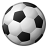# Format time calculation with hours and minutes

3396
11
11-09-2017 07:58 AMOccasional Contributor

Is there an easy way to calculate a duration from start to end displayed in hours and minutes?  I've only seen mention of either/or. I've tried a few things unsuccessfully.  Essentially I just want \${EndTime} - \${StartTime} in a h:mm format.  So, if I have StartTime = 12:00PM and EndTime = 2:06PM, I want Duration to be 2:06, not 2.1 hours or 126 minutes.

Tags (3)
1 Solution

Accepted SolutionsbyEsri Esteemed Contributor

Hi Brian,

This is pretty straightforward if a little tedious.  First to note is that time is stored internally in the Survey123 app in milliseconds (ms).  1000 ms = 1 sec = 1/60 min = 1/3600 hr.  This leads to:

# of hours = time elapsed / (3600*1000) > take the integer of the result

# of minutes (total) = time elapsed / (60*1000) > then use the modulo operation with 60 (mod 60) to get the number of minutes under a whole hour

A small thing to remember visually is to pad a zero if the number of minutes is less than 10

The Excel sheet attached steps through the formulas; all together the function (assuming the end question is t2 and the start question is t1:

concat(int((\${t2} - \${t1}) div (1000*60*60)), ":", if(int(((\${t2} - \${t1}) div (1000*60)) mod 60) < 10,"0",""), int(((\${t2} - \${t1}) div (1000*60)) mod 60))

11 RepliesbyEsri Esteemed Contributor

Hi Brian,

This is pretty straightforward if a little tedious.  First to note is that time is stored internally in the Survey123 app in milliseconds (ms).  1000 ms = 1 sec = 1/60 min = 1/3600 hr.  This leads to:

# of hours = time elapsed / (3600*1000) > take the integer of the result

# of minutes (total) = time elapsed / (60*1000) > then use the modulo operation with 60 (mod 60) to get the number of minutes under a whole hour

A small thing to remember visually is to pad a zero if the number of minutes is less than 10

The Excel sheet attached steps through the formulas; all together the function (assuming the end question is t2 and the start question is t1:

concat(int((\${t2} - \${t1}) div (1000*60*60)), ":", if(int(((\${t2} - \${t1}) div (1000*60)) mod 60) < 10,"0",""), int(((\${t2} - \${t1}) div (1000*60)) mod 60))Occasional Contributor

Thanks for you reply James.  After having time to work through your spreadsheet, I realized that the calculations were step wise and not additive.  The single calculation on the last row is all I needed.  I appreciate your help.byEsri Esteemed Contributor

No worries.  If you happen to be on UTC time, this can be simplified to t2-t1 - that represents the total number of milliseconds since midnight UTC and can be used in the format-date function (you could then add/subtract the UTC offset, though this would break for times lasting longer than 24 hours).Occasional Contributor

I just updated my calculations to use Decimal Time, based on the blog post here: https://community.esri.com/groups/survey123/blog/2016/04/17/dates-and-time-in-survey123-for-arcgis. Occasionally the calculation is 1 minute off.  When there's a 1 hour difference the Unix Time calculation correctly shows 1 hour, the Decimal Time calculation shows 59 minutes. Any suggestions?

Here's my new calculation: concat(int((decimal-date-time(\${EventEndTime}) - decimal-date-time(\${EventStartTime})) * 24), ":", if(int((((decimal-date-time(\${EventEndTime}) - decimal-date-time(\${EventStartTime})) * (24*60))) mod 60) < 10,"0",""), int(((decimal-date-time(\${EventEndTime}) - decimal-date-time(\${EventStartTime})) * (24*60)) mod 60))Occasional Contributor

Moving the hour/minute calculations into the start and end time fixed the issue.  Thanks for the suggestion at the Dev Summit, James.

concat(int((decimal-date-time(\${EventEndTime} * 24) - decimal-date-time(\${EventStartTime} * 24))), ":", if(int((((decimal-date-time(\${EventEndTime} * 24 * 60) - decimal-date-time(\${EventStartTime} * 24 * 60)))) mod 60) < 10,"0",""), int(((decimal-date-time(\${EventEndTime} * 24 * 60) - decimal-date-time(\${EventStartTime} * 24 * 60))) mod 60))by Anonymous User
Not applicable

I've followed this format and this process works in the Connect app but when testing this from a browser I get a NaN:NaN for the result, which I believe is "not a number". I'm not sure what I'm missing as in the sample spreadsheet those fields were all notes, so where is it getting confused in the process that the result is not a number? Or why is it looking for a number if the field is a note/string?New Contributor II

Did you solve why it did that for the browser only? I am having the same issue?New Contributor II

Hello James. Just like the anon below, I used the same syntax you provided, and it works great on the survey 123 app but not on the browser form. I need it to work on the browser form because that is how we plan to distribute our form. Do you know what I can do to make it work for browser?New Contributor III

Hello James Tedrick

Is it possible to calculate the hours and minutes for more than 24 hours. I tried the excel sheet you've attached here, but when fill for example (2:47 PM as start and 12:32 AM as end) this will give a minus value. I wanted to know if it's possible to get values more than 24 like 50:45 as summation.